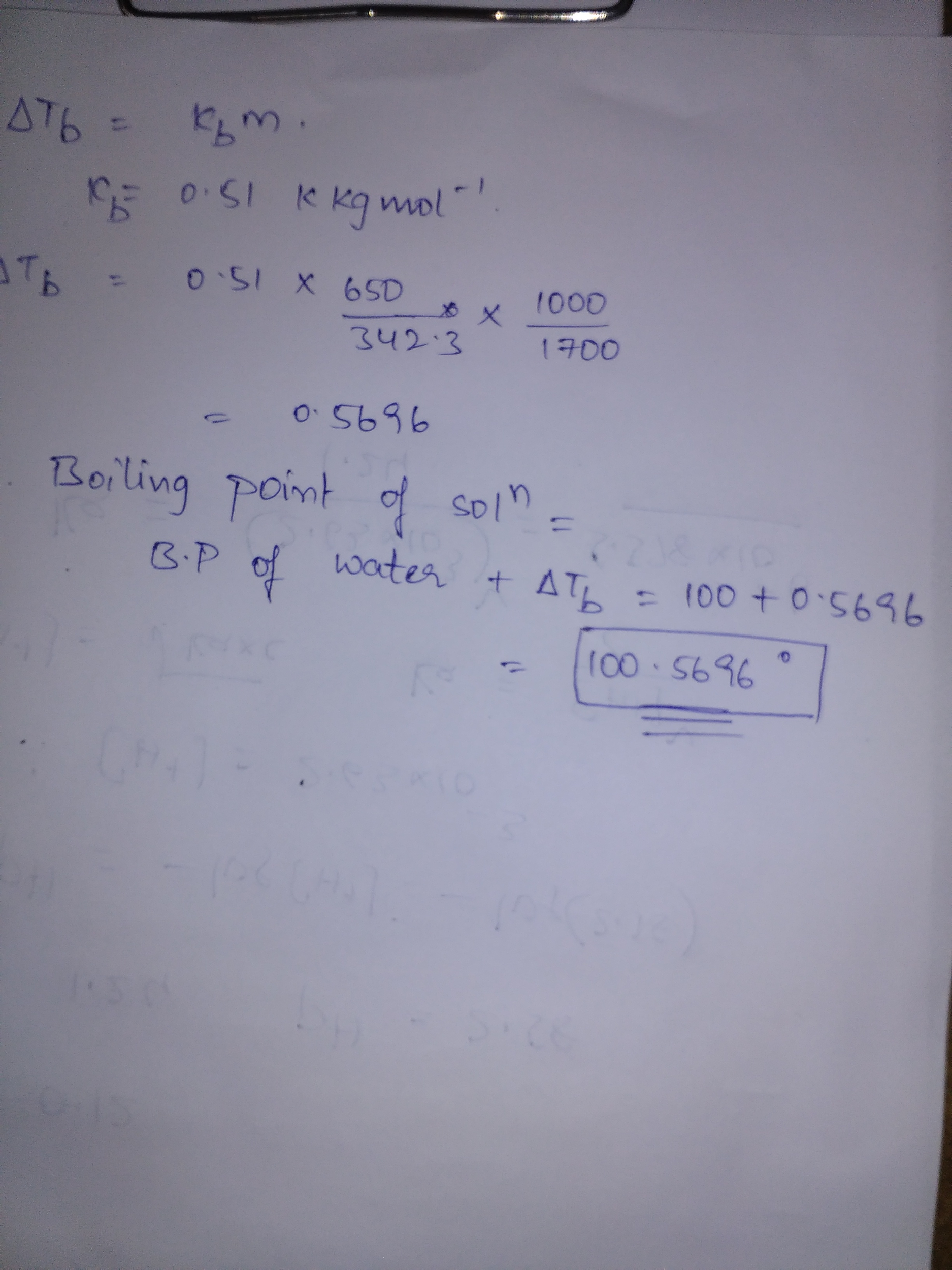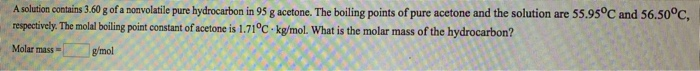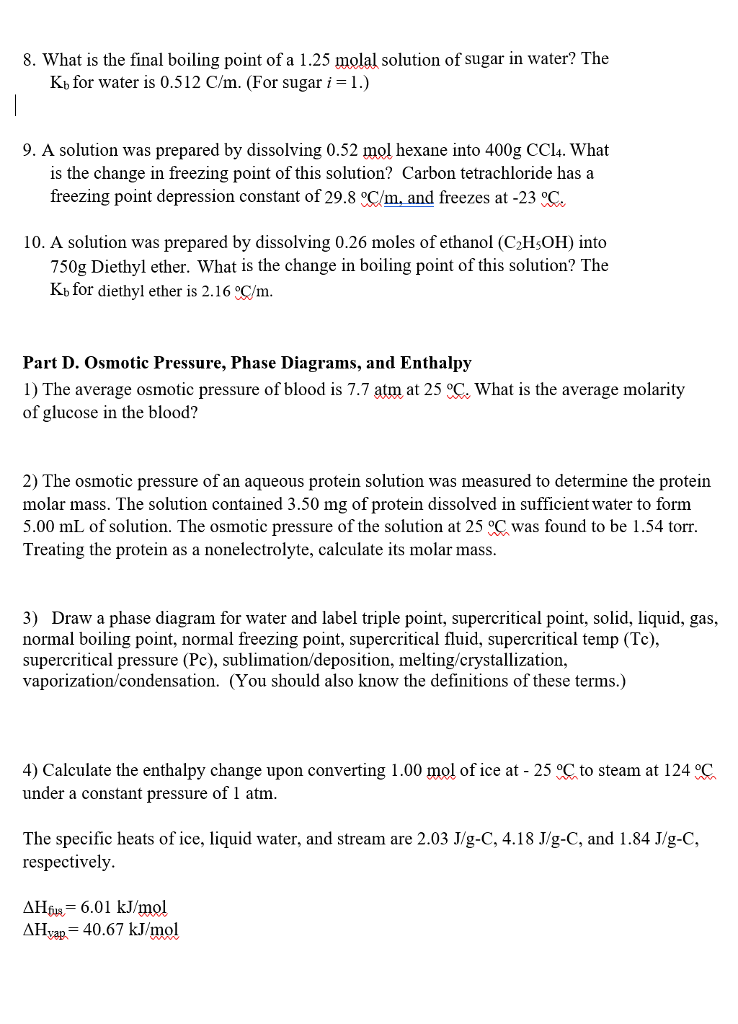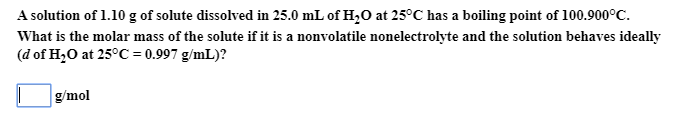Homework Help Question & Answers

# What is the boiling point of a solution produced by adding 650 g of cane sugar...

What is the boiling point of a solution produced by adding 650 g of cane sugar (molar mass 342.3 g/mol) to 1.7 kg of water? For each mole of nonvolatile solute, the boiling point of 1 kg of water is raised 0.51 ∘C.

#### Homework Answers

Answer #1Know the answer?
Your Answer:

#### Post as a guest

Your Name:

What's your source?

#### Earn Coin

Coins can be redeemed for fabulous gifts.

Not the answer you're looking for? Ask your own homework help question. Our experts will answer your question WITHIN MINUTES for Free.
Similar Homework Help Questions
• ### A solution of the sugar mannitol ( molar mass 182.2 g/mol ) is prepared by adding 54.66 g of mannitol to 1.000 kg of water

A solution of the sugar mannitol ( molar mass 182.2 g/mol ) is prepared by adding 54.66 g of mannitol to 1.000 kg of water. The vapor pressure of pure liquid water is 17.54 torr at 20o C. Mannitol is nonvolatile and does not ionize in aqueous solution. a.) Assuming that aqueous mannitol solutions behave ideally, calculate the vapor-pressure lowering for the above solution at 20o C. b.) The observed vapor-pressure lowering of the mannitol solution above is 0.0930 torr....

• ### A solution contains 0.180 g of an unknown nonelectrolyte solute in 50.0 g of water. The...

A solution contains 0.180 g of an unknown nonelectrolyte solute in 50.0 g of water. The solution freezes at –0.040 0C (notice the negative sign). A) What is the molar mass of the unknown solute? B) What is the boiling temperature of the solution? Kf(water) = 1.86 K kg mol-1   ;   Kb(water) = 0.51 K kg mol-1   . Assume that the solute only exists as a monomer in aqueous solution.

• ### A solution contains 3.60 g of a nonvolatile pure hydrocarbon in 95 g acetone. The boiling...A solution contains 3.60 g of a nonvolatile pure hydrocarbon in 95 g acetone. The boiling points of pure acetone and the solution are 55.95°C and 56.50°C, respectively. The molal boiling point constant of acetone is 1.71°C kg/mol. What is the molar mass of the hydrocarbon? Molar mass g'mol

• ### 8. What is the final boiling point of a 1.25 molal solution of sugar in water?...8. What is the final boiling point of a 1.25 molal solution of sugar in water? The Kb for water is 0.512 C/m. (For sugar i = l.) 9. A solution was prepared by dissolving 0.52 mol hexane into 400g CCl4. What is the change in freezing point of this solution? Carbon tetrachloride has a freezing point depression constant of 29.8 Cm,and freezes at-23。. 10. A solution was prepared by dissolving 0.26 moles of ethanol (C2HsOH) into 750g Diethyl ether....

• ### boiling point using molal constant

The molar mass of a nonvolatile solute is 176.0 g/mol. Compute the boiling point of a solution containing 68.0 g of the solute and 750.0 g of water, when thebarometric pressure is such that pure water boils at 99.825 °C. (Kb= 0.52 °C/m)A) 101.2 °CB) 100.1 °CC) 99.8 °CD) 98.5 °CE) 102.4 °CI keep getting B) but it tells me the answer is A)

• ### A solution of 1.10 g of solute dissolved in 25.0 mL of H20 at 25°C has...A solution of 1.10 g of solute dissolved in 25.0 mL of H20 at 25°C has a boiling point of 100.900°C. What is the molar mass of the solute if it is a nonvolatile nonelectrolyte and the solution behaves ideally (d of H,O at 25°C = 0.997 g/mL)? I g/mol

• ### An aqueous solution of a nonvolatile solute has a boiling point of 102.45 degrees Celsius. Knowing...

An aqueous solution of a nonvolatile solute has a boiling point of 102.45 degrees Celsius. Knowing that for water, Kfp = 1.86 degrees Celsius m^-1 and Kbp= 0.51 degrees Celsius m^-1. What is the molality of the solution? and what is the freezing point of the solution? Please show work.

• ### The density of a 0.84 M aqueous sugar (C12H22O11) solution is 1.12 g/mL at 25°C. What...

The density of a 0.84 M aqueous sugar (C12H22O11) solution is 1.12 g/mL at 25°C. What is the molal concentration? The molar mass of  C12H22O11 = 342.3 g/mol.

• ### What is the boiling point elevation of a solution containing 17.1 g of sucrose in 100.0...

What is the boiling point elevation of a solution containing 17.1 g of sucrose in 100.0 g of water? The molal elevation constant of water is 0.512°C/m and the molar mass of sucrose is 342 g/mol.

• ### What is the boiling point in ∘C of each of the solutions below? For water, Kb...

What is the boiling point in ∘C of each of the solutions below? For water, Kb = 0.51(∘C ⋅ kg)/mol. A solution of 15.0 g of LiCl in 158.0 g of water at 45.0 ∘C, assuming complete dissociation.

Free Homework App

Scan Your Homework
to Get Instant Free Answers
Need Online Homework Help?

Get Answers For Free
Most questions answered within 3 hours.